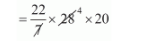# The radii of the ends of a bucket 16 cm height are 20 cm and 8 cm.`
Question:

The radii of the ends of a bucket 16 cm height are 20 cm and 8 cm. The curved surface area of the bucket is

(a) 1760 cm2

(b) 2240 cm2

(c) 880 cm2

(d) 3120 cm2

Solution:

Radius of top of bucket r1 = 20 cm

Radius of bottom of bucket r2 = 8 cm

Height of bucket = 16 cm

The curved surface area of bucket$I=\sqrt{h^{2}+\left(r_{1}+r_{2}\right)^{2}}$

$=\sqrt{16^{2}+(20-8)^{2}}$

$=\sqrt{256+144}$

$I=\sqrt{400}$

$I=20 \mathrm{~cm}$

C.S.A. of bucket

$=\pi(20+8) \times 20$$=22 \times 80$

$=1760 \mathrm{~cm}^{2}$

Hence, the correct answer is choice (a).